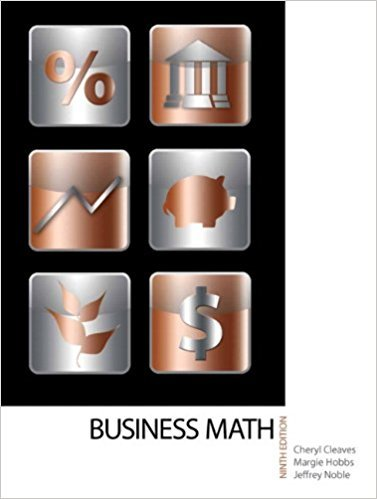×
×

# Solutions for Chapter 3-3: DECIMAL AND FRACTION CONVERSIONS## Full solutions for Business Math, | 9th Edition

ISBN: 9780135108178Solutions for Chapter 3-3: DECIMAL AND FRACTION CONVERSIONS

Solutions for Chapter 3-3
4 5 0 300 Reviews
21
4
##### ISBN: 9780135108178

Since 12 problems in chapter 3-3: DECIMAL AND FRACTION CONVERSIONS have been answered, more than 17538 students have viewed full step-by-step solutions from this chapter. Chapter 3-3: DECIMAL AND FRACTION CONVERSIONS includes 12 full step-by-step solutions. This textbook survival guide was created for the textbook: Business Math, , edition: 9. This expansive textbook survival guide covers the following chapters and their solutions. Business Math, was written by and is associated to the ISBN: 9780135108178.

Key Math Terms and definitions covered in this textbook
• Block matrix.

A matrix can be partitioned into matrix blocks, by cuts between rows and/or between columns. Block multiplication ofAB is allowed if the block shapes permit.

• Cayley-Hamilton Theorem.

peA) = det(A - AI) has peA) = zero matrix.

• Complex conjugate

z = a - ib for any complex number z = a + ib. Then zz = Iz12.

A sequence of steps (end of Chapter 9) to solve positive definite Ax = b by minimizing !x T Ax - x Tb over growing Krylov subspaces.

• Cross product u xv in R3:

Vector perpendicular to u and v, length Ilullllvlll sin el = area of parallelogram, u x v = "determinant" of [i j k; UI U2 U3; VI V2 V3].

• Elimination.

A sequence of row operations that reduces A to an upper triangular U or to the reduced form R = rref(A). Then A = LU with multipliers eO in L, or P A = L U with row exchanges in P, or E A = R with an invertible E.

• Fundamental Theorem.

The nullspace N (A) and row space C (AT) are orthogonal complements in Rn(perpendicular from Ax = 0 with dimensions rand n - r). Applied to AT, the column space C(A) is the orthogonal complement of N(AT) in Rm.

• Linear transformation T.

Each vector V in the input space transforms to T (v) in the output space, and linearity requires T(cv + dw) = c T(v) + d T(w). Examples: Matrix multiplication A v, differentiation and integration in function space.

• Multiplication Ax

= Xl (column 1) + ... + xn(column n) = combination of columns.

• Norm

IIA II. The ".e 2 norm" of A is the maximum ratio II Ax II/l1x II = O"max· Then II Ax II < IIAllllxll and IIABII < IIAIIIIBII and IIA + BII < IIAII + IIBII. Frobenius norm IIAII} = L La~. The.e 1 and.e oo norms are largest column and row sums of laij I.

• Normal matrix.

If N NT = NT N, then N has orthonormal (complex) eigenvectors.

• Orthogonal matrix Q.

Square matrix with orthonormal columns, so QT = Q-l. Preserves length and angles, IIQxll = IIxll and (QX)T(Qy) = xTy. AlllAI = 1, with orthogonal eigenvectors. Examples: Rotation, reflection, permutation.

• Projection p = a(aTblaTa) onto the line through a.

P = aaT laTa has rank l.

• Pseudoinverse A+ (Moore-Penrose inverse).

The n by m matrix that "inverts" A from column space back to row space, with N(A+) = N(AT). A+ A and AA+ are the projection matrices onto the row space and column space. Rank(A +) = rank(A).

• Rayleigh quotient q (x) = X T Ax I x T x for symmetric A: Amin < q (x) < Amax.

Those extremes are reached at the eigenvectors x for Amin(A) and Amax(A).

• Semidefinite matrix A.

(Positive) semidefinite: all x T Ax > 0, all A > 0; A = any RT R.

• Simplex method for linear programming.

The minimum cost vector x * is found by moving from comer to lower cost comer along the edges of the feasible set (where the constraints Ax = b and x > 0 are satisfied). Minimum cost at a comer!

• Singular matrix A.

A square matrix that has no inverse: det(A) = o.

v + w = (VI + WI, ... , Vn + Wn ) = diagonal of parallelogram.

• Vector space V.

Set of vectors such that all combinations cv + d w remain within V. Eight required rules are given in Section 3.1 for scalars c, d and vectors v, w.

×